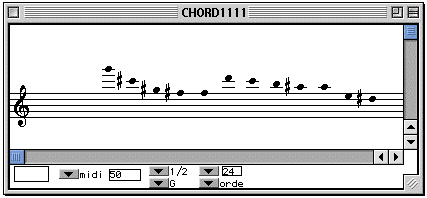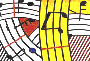Tutorial 6 - Four operations on a twelve-tone row

Topics

Recapitulation of preceeding tutorials

Functions used

CHORD, dx->x, first, om*, om+, reverse, x->dx.

Description

This patch is subdivided into four operations on the same sequence of notes (A): transposition, retrograde, inversion and retrograde of the inversion.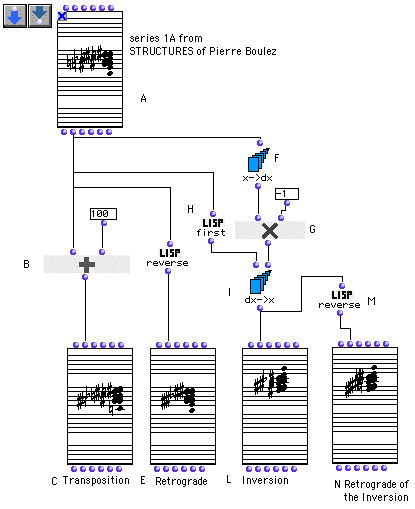Patch structure

A: Edit your series of notes in the "order" mode, close the box and and lock it.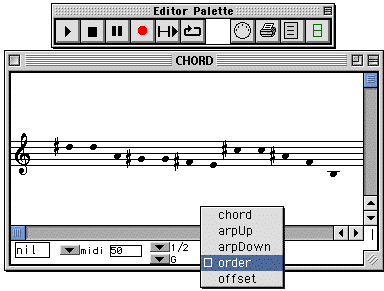Series A1 from STRUCTURES of Pierre Boulez

If you use the "order" mode the sequence is read and sent out in the order in which they were entered.

B: The om+ box transposes the sequence.

C: Evaluate and open this CHORD to see the result of the transposition.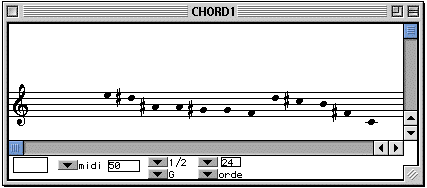D: The reverse box gives us the retrograde of the original sequence, that is to say that the notes are read from right to left.

E: Evaluate and open this CHORD-SEQ to see the result of the reverse box's operation.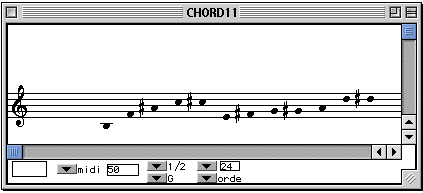F: The x->dx calculates the intervals in the original sequence of notes.

G: The module om* multiplies each interval coming out of x-dx by -
1. In doing this, it changes the sign of each interval; if we multiply 100 by -1 the result will be -100 and if we multiply -200 by -1 the result will be 200.

H: The module first takes the first note of the original series (outputting it as an atom, not as a list of one element).

I: The dx->x module creates a new sequence, starting from the first note of the original sequence (given by the first box), constructed on the intervals deriving from the om* box.

L: Evaluate and open this CHORD-SEQ to see the result.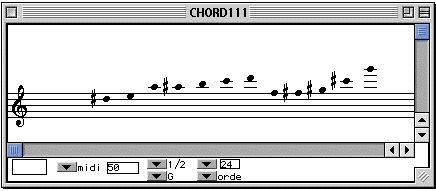M: The reverse box makes the retrograde of the sequence coming out of dx->x box.

N: Evaluate and open this CHORD-SEQ to see the result of the last operation.